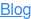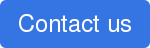# What Does Differential Reflectivity and Correlation Coefficient Tell Us

Jun 29, 2016 1:09:46 PM

The dual polarization products available in RadarScope can help provide clues in determining what really is occurring in a storm. These products can tell you about the shape, orientation, and the uniform nature of what the radar is observing. In this blog, we will look at the Differential Reflectivity and Correlation Coefficient.

Below is a reflectivity image of a severe thunderstorm, on June 27, 2016, in Northeastern Colorado. Let’s see how Differential Reflectivity and Correlation Coefficient can help determine what is falling.Dual polarization radar works on the idea that two pulses are emitted from the radar; one horizontal and one vertical. The Differential Reflectivity and Correlation Coefficient can provide information about each of these pulses. Differential Reflectivity is dependent upon the logarithm of the ratio of the power returned to the radar from the horizontal pulse to the power returned from the vertical pulse. If this ratio is greater than 1, then the feature being observed is wider than it is tall and the signal is positive; if this ratio is less than 1, the feature is taller than it is wide and the signal is negative. When the ratio is close to 1, the feature is approximately spherical. Below is a look at the Differential Reflectivity for the storm above.Large raindrops or hailstones will typically bias Differential Reflectivity. In other words, if the radar observes a mixture of rain and large hail, the Differential Reflectivity will generally mask the rain (Differential Reflectivity of Rain is usually around 1-3 dB; Differential Reflectivity of hail can range from 0 to 2 dB). In this case, the Differential Reflectivity in the heaviest echoes is around 0.9 dB.

The Correlation Coefficient is a measure of how uniform the features being observed by the radar are. It ranges in value from 0 to 1.05. Pure rain or snow should have Correlation Coefficient values close to 1. If there is a mixture of precipitation, there will be a reduction in this value. Meteorological echoes will typically have Correlation Coefficient values higher than 0.8 with non meteorological echoes (birds, insects, etc.) typically have values less than 0.8. Below is the Correlation Coefficient from this storm. Correlation coefficient values within the heaviest echoes are approximately .975.Comparing the three images above, within areas with the highest reflectivities, Differential Reflectivity values typically associated with hail are collocated with Correlation Coefficient values that are slightly less than 1. As a result, it is likely that hail is mixed in with heavy rain.## You May Also Like

These Stories on Tips & Tricks